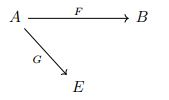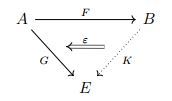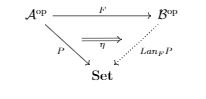# Lifting of monads to Grothendieck toposes

Published:

$\newcommand{\rv}{\mathbf{#1}} \newcommand{\x}{\rv x} \newcommand{\y}{\rv y} \newcommand{\bar}{\overline{#1}} \newcommand{\wtil}{\widetilde{#1}} \newcommand{\what}{\widehat{#1}} \newcommand{\ep}{\varepsilon} \newcommand{\ph}{\varphi} \newcommand{\maps}{\colon} \newcommand{\to}{\rightarrow} \newcommand{\xraw}{\xrightarrow} \newcommand{\darr}{\downarrow} \newcommand{\To}{\Rightarrow} \renewcommand{\dot}{\centerdot} \renewcommand{\tensor}{\otimes} \newcommand{\pr}{^\prime} \newcommand{\op}{#1^{\mathrm{op}}} \newcommand{\hom}{\textit{Hom}} \newcommand{\oo}{\circ} \newcommand{\lang}{\langle} \newcommand{\rang}{\rangle} \newcommand{\str}{#1^{*}} \newcommand{\intvl}{\mathbb{I}} \newcommand{\yon}{\mathcal{Y}} \newcommand{\topos}{\mathfrak{Top}} \newcommand{\bTopos}{\mathfrak{BTop}} \newcommand{\BTop}{\mathfrak{BTop}} \newcommand{\con}{\mathfrak{Con}} \newcommand{\pos}{\mathfrak{Poset}} \newcommand{\topl}{\mathbb{Top}} \newcommand{\grpd}{\mathbb{Grpd}} \newcommand{\ab}{\mathbf{Ab}} \newcommand{\thT}{\mathbb{T}} \newcommand{\mod}{\mathbf{Mod}} \newcommand{\th}{\mathbf{Th}} \newcommand{\cl}{\mathbf{Cl}} \newcommand{\ob}{\mathbf{Ob}} \newcommand{\aut}{\mathbf{Aut}} \newcommand{\bun}{\mathbf{Bun}} \newcommand{\geom}{\mathbf{Geom}} \def\catg{\mathop{\mathcal{C}\! {\it at}}\nolimits} \def\Cat{\mathop{\mathfrak{Cat}}} \def\Con{\mathop{\mathfrak{Con}}} \def\CAT{\mathop{\mathbf{2} \mathfrak{Cat}}} \def\Cat{\mathop{\mathfrak{Cat}}} \newcommand{\Topos}{\mathfrak{Top}} \newcommand{\ETopos}{\mathcal{E}\mathfrak{Top}} \newcommand{\BTopos}{\mathcal{B}\mathfrak{Top}} \newcommand{\GTopos}{\mathcal{G}\mathfrak{Top}} \newcommand{\Psh}{\textit{Psh}} \newcommand{\Sh}{\textit{Sh}} \newcommand{\psh}{\textit{Psh}(\cat{#1})} \newcommand{\sh}{\textit{Sh}(\cat{#1})} \newcommand{\Id}{\operatorname{Id}} \newcommand{\Ho}{\operatorname{Ho}} \newcommand{\ad}{\operatorname{ad}} \newcommand{\Adj}{\operatorname{Adj}} \newcommand{\Sym}{\operatorname{Sym}} \newcommand{\Set}{\operatorname{Set}} \newcommand{\Pull}{\operatorname{Pull}} \newcommand{\Push}{\operatorname{Push}} \newcommand{\dom}{\operatorname{dom}} \newcommand{\cod}{\operatorname{cod}} \newcommand{\fun}{\operatorname{Fun}} \newcommand{\colim}{\operatorname{Colim}} \newcommand{\cat}{\mathcal{#1}} \newcommand{\scr}{\mathscr{#1}} \newcommand{\frk}{\mathfrak{#1}} \newcommand{\bb}{\mathbb{#1}} \newcommand{\CS}{\mathcal{S}} \newcommand{\CE}{\mathcal{E}} \newcommand{\CF}{\mathcal{F}}$

Warning: These notes are severely incomplete! Hopefully I will finish them soon.

Abstract: Suppose $T: \cat{C} \to \cat C$ is a monad on $\cat{C}$. We are intereted in answering the question that under which conditions on $\cat{C}$ it is possible to lift $T$ to $\psh{C}$ and to $\sh{C}$. In order to answer this question, in this post I am going to highlight some of relevant defintions and results that are elaborated in my notes. For more details of proofs and more examples have a look there.

### What are Kan extensions?

Suppose for a $2$-category $\frk{M}$, $1$-cells $F$ and $G$ are given as follows.The question: Is there any $1$-cell $K$ such that $K \oo F =G$ ?

The answer to this depends on the structure of $2$-category $\frk{M}$. But, generically the answer is no. Take the mother $2$-category $\Cat$ of categories. Take two distinct morphisms $g$ and $h$ in $A$ in such a way that $F(g)= F(h)$ but $G(g) \neq G(h)$. Any other functor $K$ will preserve the equality of $F(g)$ and $F(h)$. On the other hand, $G$, which preserve ditinction of $g$ and $h$, cannot be written as composition of functors $F$ and $K$.

Okay, the first attempt to find a solution $K$ for filling the diagram above has failed. But, do not worry! We can use the $2$-cells in our $2$-category $\frk{M}$ to find the best possible approximation to such a solution. This approximation can be achieved from two different directions; left and right. And this is the basic idea of Kan extension.

A right Kan extension of $F$ along $G$ is the following data:

1. a $1$-cell $K: B \to E$
2. a $2$-cell $\ep: KF \To G$

univeral among such data.Note that such a $1$-cell $K$ must be unique up to unique isomorphism. Hence we denote it by $Ran_{F} G$

Note: In the case that the $2$-category $\frk{M}$ is poset-enriched we get familiar cancellation rule corresponding to the right Kan extension:

$\Updownarrow \frac{KF \leq G}{K \leq Ran_{F} G}$

And for the left Kan extension, we get:

$\Updownarrow \frac{G \leq KF}{Lan_{F} G \leq K}$

Remark: Limit of a diagram $G: \cat{A} \to \cat{E}$ is obtained as the right extension of $G$ along the unique functor $!: \cat{A} \to 1$. Similarly, colimit of a diagram $G: \cat{A} \to \cat{E}$ is obtained as the left extension of $G$ along the unique functor $!: \cat{A} \to 1$.

Remark: $\lang Lan_{F} G, \eta \rang$ is the initial object in the comma category $G/\str{F}$, and $\lang Ran_{F} G, \ep \rang$ is the terminal object in the comma category $\str{F}/G$

Let $F: \cat{A} \to \cat{B}$ be a functor. For any other category $\cat{E}$, define the functor $F^{\ast}: \fun(\cat{B}, \cat{E}) \to \fun(\cat{A}, \cat{E})$ by precomposition with $F$.

Proposition: $F^{\ast}$ has a right adjoint if and only if the right extensions of functors $G: \cat{A} \to \cat{E}$ along $F$ exists for all $G$.
Moreover, the evaluation of this right adjoint at $G: \cat{A} \to \cat{B}$ yields $Ran_{F} G$.

Similarly, $F^{\ast}$ has a left adjoint if and only if the left extensions of functors $G: \cat{A} \to \cat{E}$ along $F$ exists for all $G$. Moreover, the evaluation of this left adjoint at $G: \cat{A} \to \cat{B}$ yields $Lan_{F} G$.

### Left Kan extension and free cocompletion

Let $P$ be a presheaf on a category $\cat{A}$. We would like to compute the left extension of $P$ along $\op{F}$ (given above).In the first step, we do this computation for the easier case of representable presheaves. It is proved in the notes that $\lang \yon_ {Fa}, F_{-,a} \rang$ is a left Kan extension of $\yon_a$ along $\op{F}$. (Note: functions $F_{x,a}: \hom(x,a) \to \hom(Fx,Fa)$ for all $x$,$a$, are part of defintion of functor $F$.)

We know that any presheaf is a colimit of representables over its category of elements. Now, one can show that $Lan_{\op{F}} P$ is given by $\colim \Big(\int_{\cat{A}}P \xraw{\pi} \cat{C} \xraw{F} \cat{D} \xraw{\yon} \psh{D} \Big)$.

Since $Lan_{\op{F}} P$ exists for every presheaf $P$, $Lan_{\op{F}}$ is a left adjoint to $\Big({\op{F}}\Big)^{\ast}$ by an earlier remark. We also use the notation $F_{!}$ for $Lan_{\op{F}}$.

### Yoneda embedding as a natural-2-transformation

There is a beautiful description of yoneda embedding that goes back at least to Notions of computation and monads as far as I’m concerned. First, let’s observe that $\Psh : \catg \to \Cat$ is a $2$-functor which sends a category $\cat{C}$ to $\psh{C}$, a functor $F: \cat{C} \to \cat{D}$ to $F_{!}$ and a natural transformation $\theta: F \To G$ to $\theta_{!}$. (try to figure this out!). Note that $\catg$ is the $2$-category of small categories and $\Cat$ is the meta-$2$-category of categories.

There is also inclusion $2$-functor $inc: \catg \to \Cat$. You can see in the notes that the Yoneda embedding is a 2-natural-transformation $\yon : inc \to \Psh$.

A monad $T$ is exactly a lax functor $\ast \to \catg$. Post-composing this lax functor with $\Psh$ yields another lax funcotr which is the monad $T_{!}$; a lifting of monad $T$ to the presheaf category.